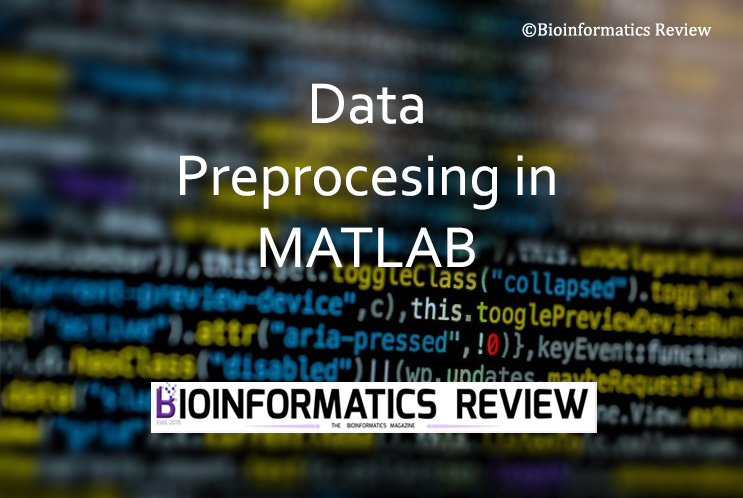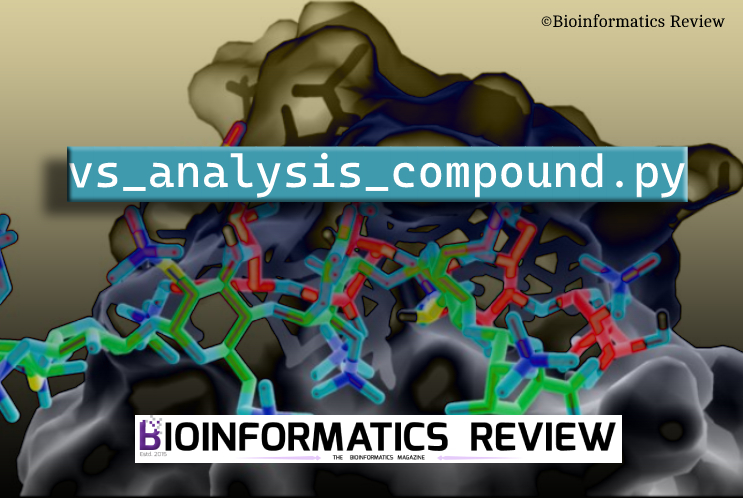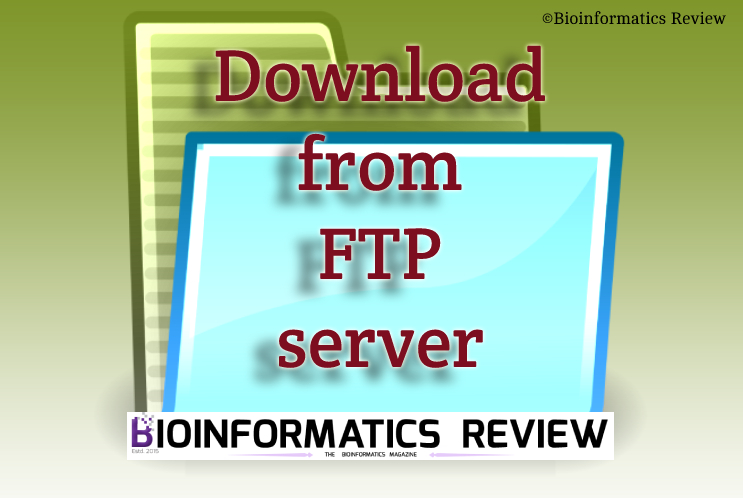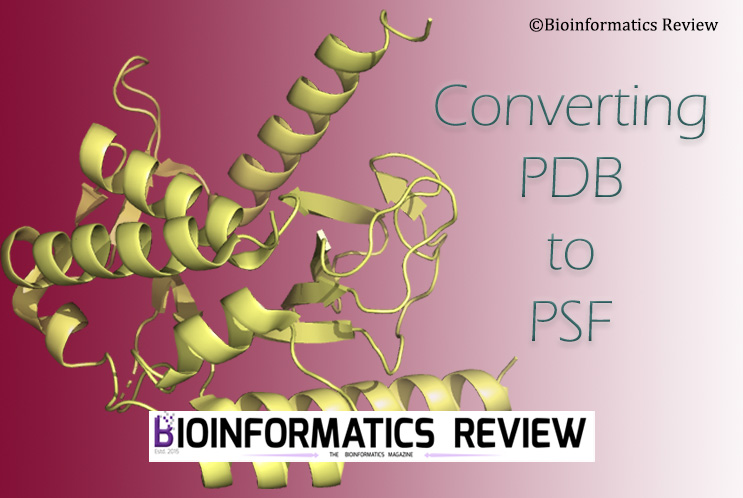# How to preprocess data for clustering in MATLAB?Published

onData preprocessing is a foremost and essential step in clustering based on machine learning methods. It removes noise and provides better results. In this article, we are going to discuss the steps involved in data preprocessing using MATLAB .

We are using sample data in the form of a CSV file consisting of 4 columns as features and 10 rows. This data consists of information about the employees working in a company including their location, age, salary, and experience. You will find some missing values there which we are going to deal with in this tutorial. Complete code is provided in the last.

### Steps in data preprocessing

1. #### Missing values

At first, you have to deal with the missing values in your data. You have three ways to do that:

i) Delete rows/columns containing missing values. You can either simply delete them or delete them based on the relative percentage of missing values. For example, if there are more than 3 values, then delete the rows/columns.
ii) Using mean. You can replace the missing values with the mean of the row/column.
iii) Using median. You can also fill in the missing values based on the median of the column/row.

2. #### Deal with non-numeric data

The non-numeric data must be taken care of before subjecting to clustering. For example, in our sample data, we have ‘Location‘ as a non-numeric feature. We will have to convert it into numeric values.

3. #### Deal with outliers

The next step is to remove the outliers from the dataset so that they cannot affect the final clustering. It can also be done in two ways as same as for the missing values either by deleting the complete row/column or by replacing the values with mean or median.

4. #### Feature scaling

Feature scaling is important because we may have different kinds of features or variables in our dataset. For instance, we have different features, such as location, age in years, salary in rupees, and experience in years. There are two ways to do the feature scaling:
i) Standardization
If you have pretty similar values then you can standardize your data. Otherwise, go for normalization. However, this is not a necessary condition, you can choose any method.
ii) Normalization

5. #### Store preprocessed data

The preprocessed data is stored in the form of a table with a variable name. This data will be further used for clustering.

Now, let’s perform these steps on our sample data one by one.

## Preparing the code

If you look at provided script right now, the first command is ‘clear’. This is to clear the workspace before running the script.

Read the data table using the ‘readtable’ command and store it in a variable.

`clear;`

`data = readtable('data.csv')`

### Dealing with missing values

#### Mean Method

As explained above, in this method we replace the missing values using the mean of the column. For example, for the Age column, we can do the following.

```M_Age = mean(data.Age, 'omitnan');
U_Age = fillmissing(data.Age, 'constant',M_Age);
data.Age = U_Age;```

Here, the missing values are replaced with the mean of Age.

Similarly, you can do for the rest of the columns except the ‘Location’. We will deal with it later.

#### Median Method

As explained above, in this method we replace the missing values using the median of the column. For example, for the Age column, we can do the following.

```M_Age = median(data.Age, 'omitnan');
U_Age = fillmissing(data.Age, 'constant',M_Age);
data.Age = U_Age;```

Here, the missing values are replaced with the mean of Age. We are using the mean method for missing values in our code.

#### Delete Method

You can delete rows or columns containing missing values. This method is not generally used, especially when having a small dataset. Therefore, we are using the mean method in our code. But you can use the following command if you wish to use the delete method for your data.

```missing_data = rmmissing(data); #for row removal containing the missing data.
missing_data = rmmissing(data,2); #for column removal
missing_data = rmmissing(data,1); #for row removal
data = missing_data;```

#### Deleting based on the relative percentage

We can also delete the rows or columns based on the relative percentage of missing values.

```missing_data = rmmissing(data,'MinNumMissing', n); #for row removal
data = missing_data;```

Here, n is the number of missing values. For example, 3, if there are 3 missing values then remove the complete rows.

### Dealing with non-numeric values

For non-numeric data such as ‘Location’ in this example, we can replace the country names based on their frequency. We can use the dummyvar function for that. It will split the names of countries into different columns and put values based on their occurrences. For instance, if the US is occurring three times, then there will put 3 under the US column.

```location_data = categorical(Location);
D = dummyvar(location_data);
```

### Outliers

Now, let’s remove outliers from our data. You can either delete rows containing outliers or replace them with mean.

#### Deleting rows with outliers

```outlier = isoutlier(data.Age);
data = data(~outlier,:);
```

#### Replacing outliers with mean

```Age = filloutliers(data.Age,'clip','mean')
data.Age = Age;
```

Similarly, you can do this for all columns in your data.

### Feature Scaling

For feature scaling, you can either do standardization or normalization.

#### Standardization

```stand_age = (data.Age - mean(data.Age))/std(data.Age)
data.Age = stand_age;
```

Similarly, do that for all columns in your data.

#### Normalization

```normalize_age = (data.Age - min(data.Age)) / (max(data.Age) - min(data.Age))
data.Age = normalize_age;
```

It depends on you how you would like to scale your data.

### Storing preprocessed data

The last step is to store your preprocessed data in a variable.

```writetable(data,'preprocessed_data.csv');
```

Now, let’s look at the code with different methods.

`clear;`
%% ————— Importing the dataset ———————-
`data = readtable('data.csv');`
%% —————Data Preprocessing —————————–
% ————– Handling Missing Values ————————
% ————– Method: Using Mean ————————–
```M_Age = mean(data.Age, 'omitnan');
U_Age = fillmissing(data.Age, 'constant',M_Age);
data.Age = U_Age;

```
% ————– Method: Using Median ————————–
```M_Age = mean(data.Age, 'omitnan');
U_Age = fillmissing(data.Age, 'constant',M_Age);
data.Age = U_Age;```

% ————– Method: Deleting rows or columns————–

```missing_data = rmmissing(data)
missing_data = rmmissing(data,2)
data = missing_data;```

% ————– Method: Deleting rows or columns based on Relative Percentage——–

```missing_data = rmmissing(data,'MinNumMissing',3);
missing_data = rmmissing(data,2,'MinNumMissing',2);
missing_data = rmmissing(data,2,'MinNumMissing',3);
data = missing_data;

```

%% ————– Handling Outliers——————————-
% ————– Method: Deleting Rows ————————

```outlier = isoutlier(data.Age);
data = data(~outlier,:);```

% ————– Method: Filling Outliers ———————

```Age = filloutliers(data.Age,'clip','mean')
data.Age = Age;```

%% ————– Feature Scaling ——————————-

% ————– Method: Standardization ———————-

```stand_age = (data.Age - mean(data.Age))/std(data.Age)
data.Age = stand_age;```

% ————– Method: Normalization ————————

```normalize_age = (data.Age - min(data.Age)) / (max(data.Age) - min(data.Age))
data.Age = normalize_age;```

%—————-Storing preprocessed data———————–

`writetable(data,'D:\preprocessed_data.csv');`

We have explained for one column only. You can include all columns in the respective sections of the code. Save this file as preprocessing.m and then keep the data.csv in the same directory or provide a full path in the script, and then run it.

#### References

1. MATLAB and Statistics Toolbox Release 2022b, The MathWorks, Inc., Natick, Massachusetts, United States.Dr. Muniba is a Bioinformatician based in New Delhi, India. She has completed her PhD in Bioinformatics from South China University of Technology, Guangzhou, China. She has cutting edge knowledge of bioinformatics tools, algorithms, and drug designing. When she is not reading she is found enjoying with the family. Know more about Muniba

# vs_analysis_compound.py: Python script to search for binding affinities based on compound names.Published

onPreviously, we have provided the vs_analysis.py script to analyze virtual screening (VS) results obtained from Autodock Vina. In this article, we have provided another script to search for binding affinity associated with a compound. (more…)

# How to download files from an FTP server using Python?Published

onIn this article, we provide a simple Python script to download files from an FTP server using Python. (more…)

# How to convert the PDB file to PSF format?Published

onVMD allows converting PDB to PSF format but sometimes it gives multiple errors. Therefore, in this article, we are going to convert PDB into PSF format using a different method. (more…)